﻿ The Metrological Assurance of the Colorimetry in Software and Hardware Environments
Journal of Telecommunications System & Management
All submissions of the EM system will be redirected to Online Manuscript Submission System. Authors are requested to submit articles directly to Online Manuscript Submission System of respective journal.
• Research Article
• J Telecommun Syst Manage 2016, Vol 5(3): 146
• DOI: 10.4172/2167-0919.1000146

# The Metrological Assurance of the Colorimetry in Software and Hardware Environments

Yauheniya Saukova* and Matyush I
Department of Standardization, Metrology and Information Systems, Belarusian National Technical University, Minsk 220013, Belarus, USA
*Corresponding Author: Yauheniya Saukova, Associate professor, Department of Standardization, Metrology and Information Systems, Belarusian National Technical University, Minsk 220013, Belarus, USA, Tel: (+375) 296839006, Email: [email protected]

Received Date: Nov 09, 2016 / Accepted Date: Nov 22, 2016 / Published Date: Dec 02, 2016

### Abstract

This work is devoted to the issues of colorimetric measurements in hardware and software environments using technologies of digital image processing. Such measurements can be implemented by ensuring metrological traceability of results to standards or reference materials. The main idea of the research is to model and build a reference scale in a functional vector color space in space by creating a set of measures (reference samples) sources. To select the color space XYZ on sectors and build in each sector the calibration characteristics is proposed in this paper. This procedure allows to reduce the methodical component of uncertainty and to do measurements in hardware and software environments.

Keywords: Metrological assurance; Colorimetry; Environment; Photometric; Normalization

#### Introduction

An advanced level of hardware and software development allows using the digital images as informational objects models for research of their photometric and colorimetric properties. Such research is relevant in the simulation of the light environment and objects state monitoring at all stages of their life cycle. However, for realization of measurements it is necessary to adhere to the principle of comparison with a measure. This is a problem because there are many difficulties with ensuring of the metrological traceability of results, given the diversity of hardware and software, as well as the use of computer ranking scales for the measurement of the intensities of digital images. In addition, the differences and limitations of transmitting devices hamut prevent the obtaining of reliable measuring information. Solutions to these problems are proposed in this paper, which will allow carrying out the colorimetric measurements in hardware and software environments. The main attention is concentrated on the idea of constructing a reference scale in CIE XYZ  space based on the material and the virtual reference samples (measures).

#### Materials and Methods

In the working process on the project both theoretical and experimental research methods based on investigation of the Scientific Research Laboratory of Optoelectronic Instrumentation (Belarusian National Technical University, Minsk, Belarus) and leading lighting laboratories of Belarusian State Institute of Metrology, National Academy of Sciences of Belarus, etc. are used. Standard samples (national standard of chromaticity coordinates and created certified palettes and led displays) were used in the experiments. Results of investigations suggest that the High Resolution Colorimetric can be applied to extended objects. Implemented the accuracy of photometric and colorimetric measurements results is ±10%. The developed methods are the basis for a methodology for measuring, registration and visual objects control using digital images. This methodology may be applicable to validation and verification of registration, display devices and test methods in accredited laboratories.

The color as a measured and its interpretation in functional spaces

There are nine Statute definitions of a color at present which distort the visual perceptions. We can distinguish three basic definitions among them. According to  “a color is an affine vector quantity of three dimensions expressing a property common to all spectral compositions of the radiation, visually indistinguishable in the colorimetric conditions of observation”. According to  “the color (perceived) is a property of visual perception, combining chromatic and achromatic features”. There are else six definitions in this document. In accordance with  “color is uniquely characterized for a certain specific observer and light source by coordinates of the point in vector space”. Thus color is a vector quantity and can be described by standardized functional spaces, based on empirical models of standard observer perceptions and regulated by ISO 11664-1 .

The model CIE 1931  developed by Wright and Guild and based on color perceptions research of 17 respondents in the wavelength range from 400 to 700 nm with the addition of a mixture of substances of red, green and blue, for corner 20 degrees looks like this: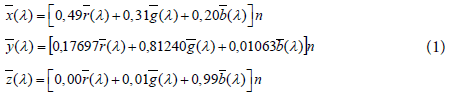Where,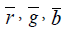functions that have been received as an identical spectral efficiency of CIE;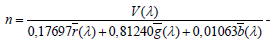. The normalized constant function.

Model CIE 1964, developed by Stiles, Barham and Speranskaya on research on 67 of observers, based on monochromatic stimuli by fitting a spectrum from 390 to 830 nm with the addition of the proportions of R, G, B light fields using a field of view 100 (not ignoring the Central 40 or so) :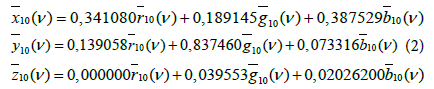There are many other models in the literature  sophisticated of color perception - Nayatani, hunt, RLAB, CIECAM97s, CIECAM02 and etc. They take into account the spatial and temporal characteristics of perception to predict a wide range of visual phenomena by introducing functions of chromatic adaptation of the visual analyzer and technical devices. However the typical tasks of measuring control are handled in standard conditions. Therefore special attention will be paid to applied families spaces RGB and CIE XYZ in this paper. Hardware dependent space ISO RGB, sRGB, ROMM RGB, Adobe RGB 98, Apple RGB, video RGB are described in detail in  and their comparative analysis is presented. These spaces are used to represent the color intensities and hamut soft rendering devices.

As a rule the point of black color serves as the origin. The selection of main radiations, directions of the axes, magnitude is arbitrary and depends on goal . A portion of the color space with primary colors (red-R, green-G, and blue-B) is shown on Figure 1. The expansion or contraction of the color space occurs due to the modification of angles between the coordinate axes. Axis come from one point and doesn’t lie in the same plane.

A plane is drawn through the coordinates of these basic cuts an equilateral triangle. An achromatic color vector passes through 1/3 of the height of this triangle. The direction of the vector indicates the increasing lightness (Figure 2a). Any other vector that is not coincident with the achromatic vector reflects not only the brightness but also chromaticity. If the color vector is closer to any one axis the saturation and color tone will be more pronounced. Single colors differ in brightness. Single colors can be the same brightness if you find them appropriate proportions. However not all primary colors will be in the plane of a single color, they also belong to the plane of equal brightness (Figure 2b). The plane of equal brightness is carried out through the ends of new vectors and intersects with the single plane along a straight line of equal brightness. This implies that some individual colors belong to two planes and have the same brightness. We can stress a lot of lines (and parallel planes) of equal brightness. The primary colors in the RGB-system are real and curves of the addition which were obtained experimentally and calculated describing the spectral sensitivity of the eye were used for the construction of systems of the second type-such as the XYZ-space .

Color gamut the visual apparatus of man is the cross-section color-a new space plane for the single colors (Figure 3) .

Figure 3: The position of a locus of spectral colors relative to the triangle a single color.

Due to Grassmann’s law there is a linear dependence between any coordinates of colors expressed in different systems :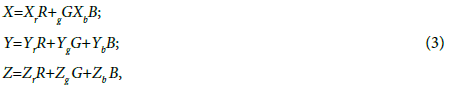Where Xr, Yr, Zr-a coordinate R, expressed in XYZ system;

Xg, Yg, Zg-a coordinate G, expressed in XYZ system;

Xb, Yb, Zb-a coordinate B, expressed in XYZ system.

We can build infinite multitude of such coordinate systems. It is necessary to use basic radiations of increased saturation that greater than the spectral colors to obtain only positive of the color coordinates. In this case all spectral colors will be the colors of a single triangle. The authors took as a basis one of the type XYZ-systems to illustrate the proposed concept because this system is most frequently used in the inspection and test of products (Figure 4).

Figure 4: The part of absolute CIEXYZ-space with a triangle and a single colors and the locus of spectral colors (E- the coordinates of the source with light of equal energy).

However the normalized color space xy Y are more frequently used in practice. Normalization is performed by transferring the color coordinates X, Y, Z the chromaticity coordinates x, y, z as follows [8,9]: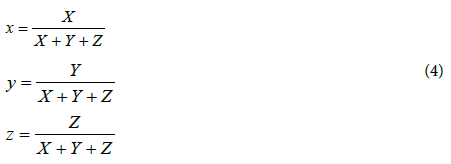Then new color space points are chosen from the entire set to satisfy the condition of belonging to the chromaticity triangle: x+y+z=1. The coordinate z describes the lightness affecting the education of shades . The arbitrary choice of scales for the coordinate axes leads to a change in the shape of the color triangle and the relative positions of points color grade. In this regard the concept of length loses its meaning in the color space since the scale factors linking the modules of color and brightness in different directions are different. This space unlike Euclid’s is called affine. In affine space the concept of parallel lines and planes is preserved but the ratio of lengths and angles for non-parallel lines are not preserved .

The basic concept of the measurements

There are many difficulties arise in solving measurement problems associated with determining the luminance and color characteristics of objects based on processing their digital images. These difficulties are associated with objective limitations of color coverage, and almost all economic ranges of the transmission device, and establishing the corresponding nominal level of quantization in the color channels as a result of the normalization of the digital image at the brightest point. These problems can be solved by the correct application of available hardware and software according to the proposed method of measurements. The method is based on digital registration of objects with different values of exposure time and digital images processing which the transformation of color spaces RGB→XYZ is in using a special introduced expansion coefficients allowing with the given confidence level to determine the color characteristics of the studied . Metrological traceability of measurement results is provided by reference to the initial samples (called by authors as a line sources with equal brightness) which are non-point sources of light acting as the measures in measurements. Each line is formed on the basis of belonging to the spectral composition of selected sector radiation of the specific standardized color palette data is stored in special tables of support software. The example of a palette is shown on the Figure 5.

The authors offer to divide the palette into six sectors according to the principle of precedence of the color coordinates specific weights (in descending order of intensity in the color channel digital images): 1) RGB; 2) RBG; 3) GRB; 4) GBR; 5) BRG; 6) BGR. This operation allows reducing the area of the methodical component of uncertainty.

Within each of the line light sources should vary in brightness with a step depending on the specific measuring task. Thus we can construct a set of calibration curves for each source showing the dependence of intensity values (y-axis) of the color channels from the exposure time (x-axis)-R(t), G(t), B(t), an example of which is given in Figure 6. Further according to mated for each consecutive pairs of sources for example one line for one time. The values of intensities of R, G, B for exposure time’s tm pending solid lines in the figure on the y-axis

As can be seen from Figure 6, in the red channel, the intensity of Rn lies in the region of saturation. This is due to limitations in the dynamic ranges of digital cameras and display devices and therefore, makes it impossible to obtain accurate measurement information. The offset of intensity in the green channel G in the area of noise may occur when switching to the image with exposure time tm-1<tm. Therefore to base on the principle of linearity of the calibration characteristics of the measurement method we can determine on the y-axis conditional point R′n outside dynamic range and carry out the normalization of the intensities of R, G, B, adapting them to new values, introducing appropriate broadening coefficients directly in a standardized model transformation color spaces RGB→XYZ :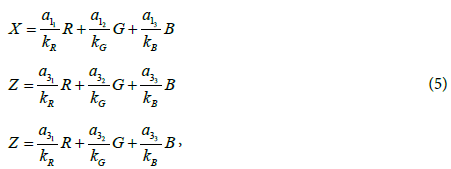where X, Y, Z-coordinates of the color space XYZ;

anm -the standardized weighting of intensities R, G, B;

kR, kG, kB – expanding the coefficients calculated by the formulas: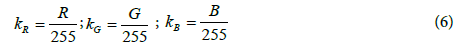The highest value of the digital intensity image is given in the denominators (there is 255 gradations of intensity in this case). New transformed lines of intensity in the color channel and G by the stipple. The calculations of the chromaticity coordinates produced by the formulas (4). Thus it was established experimentally that color coordinates triads of the same reference point on the object in images made with different exposures with a given confidence level correspond to the same Chroma. It was also found that the greatest variance of the intensity values observed in the blue channel. The averaged variance of determination of chromaticity coordinates is from 2.8 to 3.1 units approximately. Reliable detection of detected object real color coordinates depends on the nominal values of quantization steps, playing required scales conventional digital image. The nominal level of quantization represents the ellipsoids of different volumes in threedimensional color spaces, and the minimum (definitely) uncertainty ellipses are determined by the Mac-Adam. However the constant value of the nominal level of quantization can be maintained in the RGB color space by the mathematical methods of the increment intensity. There is a possibility to define the color characteristics of self-luminous objects and to enter correction factors for secondary emitters by applying models of chromatic adaptation Von-Crease, CIECAM02, Fairchild and others. The color coordinates are defined in the vertical section of space XYZ (the coordinate Z) by normalization of the original samples intensity. The authors suggest taking digital images of an object from one point of an environment with an increasing exposure time. After that it is necessary to determine the brightness of a control point of the digital image in the three color channels through computer processing, compare it with reference values and deter-mine the color coordinates and the chromaticity of a given point in the coordinates of the standard color spaces, for each (red, blue and green) color channel according to the formula :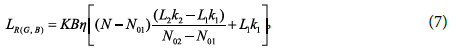Where K- the scaling factor;

B-a factor depending on the spectral properties and the scattering phase function at the reference point of the object surface;

η-a coefficient taking into account the registration parameters;

N-the output signal of the CCD matrix corresponding to the brightness control point in the image object for the channel, expressed in relative units;

L1, L2 -the reference brightness of the color reference targets in this color channel, cd/m2;

N01, N02-output signals of CCD-matrix corresponding to the intensity of the image of the color target in this color channel.

Data conversion must be performed for each color channel. It should be consider surface properties (reflectance) and parameters of shooting in the calculations. The Figure 7 explains the essence of the developed model. Different signals N’01, N’02, N’’01, N’’02, etc. correspond to the reference brightness values L1, L2 on the abscissa. The linear dependences can be built on control points of the photo detector dynamic range before the transition to the stage of saturation. By pairing the dependency mathematically with sufficient accuracy for practice it is possible to calculate intensity values of points on the object in three color channels, thus broadening the range of two-dimensional colorimetric measurements.

Figure 7: The extension of detected brightness dynamic range of the images made with different exposures (t1<t2<t3).

Preliminary calculations: The transformation RGB→XYZ →xyz

For realization of measuring procedure it is necessary to provide principle of comparison of measured with its unit or measure. In the colorimetric of high resolution it is achieved by the construction of some conventional scale, the stages of that would ensure metrology traceability to the standard sample. Assuming as standard samples may be used non-point chromatic light sources uniformly distributed over the surface brightness (for example, colored flat LEDs) then on the basis of attested values of brightness characteristics can construct graduation curves. For this purpose it is proposed on the basis of numerical modeling to establish calibration curves display patterns in the space XYZ. In zoned standard color palette has been taken as an example to preliminary calculations, shown in Figure 6. To construct the calibration curves in the space XYZ color values have been chosen coordinates lying at the boundaries of each of the sectors obtained (Figure 5). For the coordinate conversion in RGB coordinates XYZ :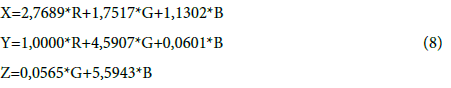Next it is indispensably to calculate the chromaticity coordinates by the formulas (4). The calculation results in the form of projections points on the globe and are shown in the Figure 2a. However, interest is the border between the sectors I to VI (Figure 2b). The results of color coordinate conversion at the boundaries of each of the six sectors are shown in Figure 8.

Roman numerals from I to IV are indicated sectors corresponding to the sectors in Figure 9.

Figure 9: The results of conversion of coordinates of points of the calibration curves.

As a result of the construction of the points obtained by the coordinates we have divided into 6 sectors locus in the xy plane (Figure 10).

The principle of conditional reference scale constructing

Consider the technique of finding the numerical value of a luminous object characteristic (we assume that the measurements object) as the power light. The color of the host color space (the object) is represented by a point with coordinates (x; y; z), in which the vector is directed from its origin. We are interested in the direction along the axis oz, because along it color vectors are arranged in this way that at least the white falls on top of it, and up to a place where there is a point E (the so-called white point) as shown in Figure 11. This vector will be used as a so-called binding.

We will find the numerical value of the light intensity using the calibration straight line constructed in the coordinates (L; N), where L - luminous intensity in candela (cd), and the N - axis along which the values obtained by calculation will be located, (%) (Figure 12).

Previously it is known that this space is divided into six sectors (see point 2), according to the standard RGB color palette (Figure 12). We assume that for each of them (sectors) selected pair of certified standard samples of light sources, of which we know the values of all the characteristics of interest, including the numerical values L1 and L2 of the light power. Data samples are selected such that their positions in the modeling color vector in the XYZ color space to the coordinate axis vectors of the inequality:

z1< zn < z2;

where z1 - coordinate of the color of the first sample on the 0z axis;

z2 - vector color coordinate of the second sample on the 0z axis;

zn - coordinate any other vector color standard RGB palette belonging to the same sector XYZ space, and color vectors of the first and second standard samples (Figure 13).

We consider that these samples we have: For information on the object of interest to us we get with the help of its digital processing of images obtained by the photo device. From this we know we are interested in the object coordinates of RGB pixels, which allows using the conversion formulas to get their corresponding coordinates in XYZ space and perform a clean build. However, the construction of a simple enough. One and the same object, recorded with different exposure times can have multiple implementations in the space, as shown in Figure 14.

Figure 14: The implementation of the same object in the space of a host image is obtained several times with different exposure times, 1, 2, 3, 4, 5, and 6 respectively.

The situation is illustrated in Figure 14 indicates that the register object is necessary, together with standard samples to rule out false, does not correspond to the actual location of their vectors (color vectors of the object and the two samples) in space and, as a consequence, an incorrect finding of interest to us, the light characteristics of the object.

Given this sort of variation and the fact that the color vector objects and specimens are not on the same straight line, but the same direction to get the values 0N axis (Figure 15) it is necessary to tie all three vectors to any one vector, which then we transform in 0N axis. Vector OE (Figure 14) directed from the origin to the white point is very well suited for this. We know the value of a point E coordinate belonging to this vector:

x=0.333;

y=0.333;

z=0.333.

Consequently the angle “α”, under which the vector is directed with respect to each of the axes is 45° (Figure 15).

Vector OE will use the following way: it project the vectors of the two available samples and the resulting pre-registration and conversion device digital coordinates of the vector object (Figure 16).

Further assume that the entire vector OE will have 256 divisions. You need to find a range of values of such a “scale”, which belongs to the segment on a vector OE formed between the ends of the projections of the vectors it two standard samples. (For convenience, we will refer to the points of projection vectors OE such as shown in Figure 17). The value of the desired length L will equal to the difference between the sections 2 and 1. We find them the length of the triangles ABC and ADE (Figure 17).

Figure 17: Diagram of the European Union to find the length of the segment (corresponding to the segment 1 - 2 in Figure 13).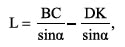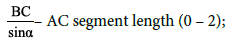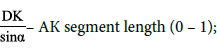The numerical values of the lengths of BC and DK will be equal to the coordinate values along the axis 0z vectors of the first and second samples, respectively.

Furthermore we are interested in the location of the segment in the vector OE. This is possible when we know the length of the projection of the first sample by a vector OE (AK segment) and a length of segment L. With given the fact that the entire vector, we were divided into 256 parts (100%), and the entire length of the, where z1(OE)- numerical value of the coordinates of the point E in the space XYZ. We get the following thing (Figure 18):

Figure 18: Finding the size and location of the vectors point in the new coordinate system.

“Axis” is replaced by “Axis”, cd- on cd

Similarly are the coordinate axes on ON object: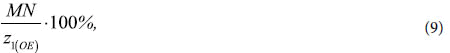where MN - segment, the value of which is equal to the numerical value of the coordinates of the z-axis of the vector object’s color;

z(OE)- interval, the value of which is equal to the numerical value of the coordinates of the z-axis of the vector OE.

As a result, we have the following formula to calculate the coordinates of sample points and the object in the new coordinate system (Figure 19).

That is, the sample light sources need to find a location for the ONaxis (how - described above), and knowing the numerical values of their intensity to construct a calibration line by means of which it is possible, knowing the object only its coordinates RGB to obtain the numerical value of its intensity, pre running the Exchange the coordinates by the already known and newly derived formulas.

The Automation of measurement procedures: Computer program description

This application is intended for use in the course of measurements, the purpose of which is to determine the characteristics of various light-emitting products.

The application can be used for measurement of physical quantities of light-emitting products in various fields of science and technology related to the production of lighting products and studies their characteristics. The software interface is shown in Figure 20.

Getting the first stage of the information about the object as its coordinates in the RGB color space to the second stage, with this application (by registering this information in the appropriate fields) at the output value of interest to us, the physical quantity. To the implementation of the second phase was the possible need to have a certain way matched certified reference materials (information about them should be presented and RGB values of the coordinates of interest to physical quantity registered operator set aside for this field). After filling out the fields “Value of measure and” to a “Sample №1» and « Sample №2» as well as «R», «G» and «B» for the standard samples and the object by pressing the “POSTROITGRAFIK” obtain the desired value of the physical values and its graphic representation in relation to the values of the physical quantity of standard samples.

Calculations and building taking place with reference to the XYZ color space. An example of an application submitted by the calculated result of the physical quantity of the object, the coordinates in XYZ space...

Interface language: English.

System requirements: Windows XP SP3, Windows Vista, Windows 7, Windows 8, Windows 10.

Processor: 400 Hz and higher.

Memory (RAM): 126 mb.

Required disk space: 7,25 mb.

#### Conclusion

It is necessary to adhere to the principle of comparison with a measure for realization of measurements. This is a problem because there are many difficulties with ensuring of the metrological traceability of results, given the diversity of hardware and software, as well as the use of computer ranking scales for the measurement of the intensities of digital images. There many models in the literature sophisticated of color perception. The typical tasks of measuring control are handled in standard conditions. We can build infinite multitude of such coordinate systems. It is necessary to use basic radiations of increased saturation that greater than the spectral colors to obtain only positive of the color coordinates. In this case all spectral colors will be the colors of a single triangle. The authors took as a basis one of the type XYZ-systems to illustrate the proposed concept because this system is most frequently used in the inspection and test of products.

Getting the first stage of the information about the object as its coordinates in the RGB color space to the second stage, with this application (by registering this information in the appropriate fields) at the output value of interest to us, the physical quantity. To the implementation of the second phase was the possible need to have a certain way matched certified reference materials (information about them should be presented and RGB values of the coordinates of interest to physical quantity registered operator set aside for this field). After filling out the fields “Value” to a “ Sample №1» and «Sample №2» as well as «R», «G» and «B» for the standard samples and the object by pressing the “to build graphic” obtain the desired value of the physical values and its graphic representation in relation to the values of the physical quantity of standard samples. Calculations and building taking place with reference to the XYZ color space. An example of an application submitted by the calculated result of the physical quantity of the object, the coordinates in XYZ space.

#### References

Citation: Saukova Y, Matyush I (2016) The Metrological Assurance of the Colorimetry in Software and Hardware Environments. J Telecommun Syst Manage 5: 146. Doi: 10.4172/2167-0919.1000146

Copyright: © 2016 Saukova Y, et al. This is an open-access article distributed under the terms of the Creative Commons Attribution License, which permits unrestricted use, distribution, and reproduction in any medium, provided the original author and source are credited.

Select your language of interest to view the total content in your interested language

##### Recommended Journals
Viewmore
###### Article Usage
• Total views: 1530
• [From(publication date): 0-2016 - Nov 12, 2019]
• Breakdown by view type
• HTML page views: 1447Can't read the image? click here to refresh